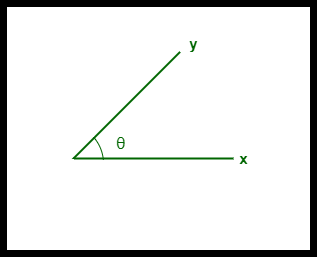Cosine Similarity

• Difficulty Level : Basic
• Last Updated : 06 Oct, 2020

Prerequisite – Measures of Distance in Data Mining

In Data Mining, similarity measure refers to distance with dimensions representing features of the data object, in a dataset. If this distance is less, there will be a high degree of similarity, but when the distance is large, there will be a low degree of similarity.

Some of the popular similarity measures are –

1. Euclidean Distance.
2. Manhattan Distance.
3. Jaccard Similarity.
4. Minkowski Distance.
5. Cosine Similarity.

Cosine similarity is a metric, helpful in determining, how similar the data objects are irrespective of their size. We can measure the similarity between two sentences in Python using Cosine Similarity. In cosine similarity, data objects in a dataset are treated as a vector. The formula to find the cosine similarity between two vectors is –

Cos(x, y) = x . y / ||x|| * ||y||

where,

• x . y = product (dot) of the vectors ‘x’ and ‘y’.
• ||x|| and ||y|| = length of the two vectors ‘x’ and ‘y’.
• ||x|| * ||y|| = cross product of the two vectors ‘x’ and ‘y’.

Example :
Consider an example to find the similarity between two vectors – ‘x’ and ‘y’, using Cosine Similarity.

The ‘x’ vector has values, x = { 3, 2, 0, 5 }
The ‘y’ vector has values, y = { 1, 0, 0, 0 }

The formula for calculating the cosine similarity is : Cos(x, y) = x . y / ||x|| * ||y||

x . y = 3*1 + 2*0 + 0*0 + 5*0 = 3

||x|| = √ (3)^2 + (2)^2 + (0)^2 + (5)^2 = 6.16

||y|| = √ (1)^2 + (0)^2 + (0)^2 + (0)^2 = 1

∴ Cos(x, y) = 3 / (6.16 * 1) = 0.49

The dissimilarity between the two vectors ‘x’ and ‘y’ is given by –

∴ Dis(x, y) = 1 - Cos(x, y) = 1 - 0.49 = 0.51
• The cosine similarity between two vectors is measured in ‘θ’.
• If θ = 0°, the ‘x’ and ‘y’ vectors overlap, thus proving they are similar.
• If θ = 90°, the ‘x’ and ‘y’ vectors are dissimilar.Cosine Similarity between two vectors